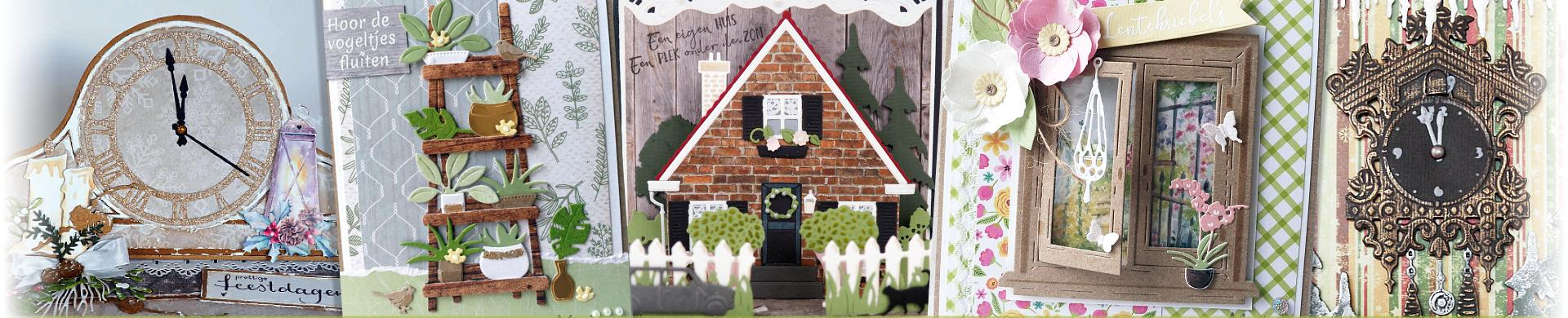# Cutting Dies - House & HomeHouse & Home themed thin metal cutting dies for card making and papercrafts.
• Brand
1. \$5.56
2. \$10.20
3. \$5.56
4. \$6.48
5. \$6.48
6. \$6.48
7. \$5.56
8. \$6.48
9. \$5.56
10. \$13.63
11. \$8.07
12. \$12.06
13. \$3.24
14. \$6.48
15. \$9.73
16. \$3.98
17. \$3.98
18. \$4.91
19. \$6.48
20. \$4.63
21. \$6.48
22. \$11.12
23. \$5.93
24. \$8.34
25. \$7.42
26. \$5.00
27. \$10.76
28. \$16.70
29. \$8.34
30. \$7.23
31. \$8.34
32. \$7.69
33. \$10.38
34. \$4.91
35. \$8.81
36. \$8.34
37. \$5.56
38. \$7.42
39. \$7.42
40. \$8.34
41. \$15.30
42. \$7.42
43. \$11.12
44. \$7.42
45. \$13.45
46. \$10.20
47. \$8.34
48. \$6.48
49. \$3.70
50. \$4.63
51. \$6.86
52. \$7.42
53. \$12.06
54. \$11.12
55. \$9.28
56. \$8.81
57. \$6.02
58. \$6.21
59. \$8.34
60. \$3.70
61. \$7.42
62. \$6.02
63. \$10.20
64. \$9.28
65. \$13.45
66. \$18.55
67. \$18.55
68. \$6.48
69. \$8.34
70. \$3.70
71. \$3.42
72. \$6.48